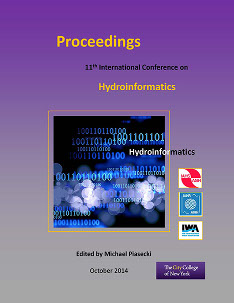## International Conference on Hydroinformatics

Presentation

8-1-2014

#### Abstract

Discharge determination in open channels is of great interest for water engineering, especially in compound channels where the main difficulty is the link between the measured velocity and the mean velocity. To do so, the 3D modeling is used as a tool to get the velocity distribution in compound channels. The purpose of this study is to compare through numerical simulations, the most common turbulence models such as K-epsilon and RSM to LES that is rarely used in this field, in order to show the efficiency of the LES model to reproduce the velocity distribution. The method used is the following: an experimental case (NEZU 1991) was modeled with RSM, k-epsilon, k-w sst and LES using two different software (Ansys Fluent® and OpenFoam®). Finally, for each model, the three parameters of the velocity U, V and W, the turbulence kinetic energy Tke and the Reynolds stress tensors were compared with the experimental case in order to identify the closest model to Nezu’s results. This research has found that for a certain limit (Re104), the RANS models coupled to Ansys Fluent® software can reproduce correctly neither the velocity distribution nor the secondary currents. This case represents a limit for the RANS models. In comparison the LES model allowed to display a good representation of velocity distribution, secondary currents and of the dip phenomenon. By using the recycling method (Castro 2011) to generate a turbulence inlet velocity, LES shows also the advantage of decreasing the number of grid cells the computational domain, which makes the computational time last less longer. Finally, contrary to the RANS model, the LES model gives information at each time step which allows the researcher to get details that are not provided by the RANS model such as the birth of turbulence in the main channel.